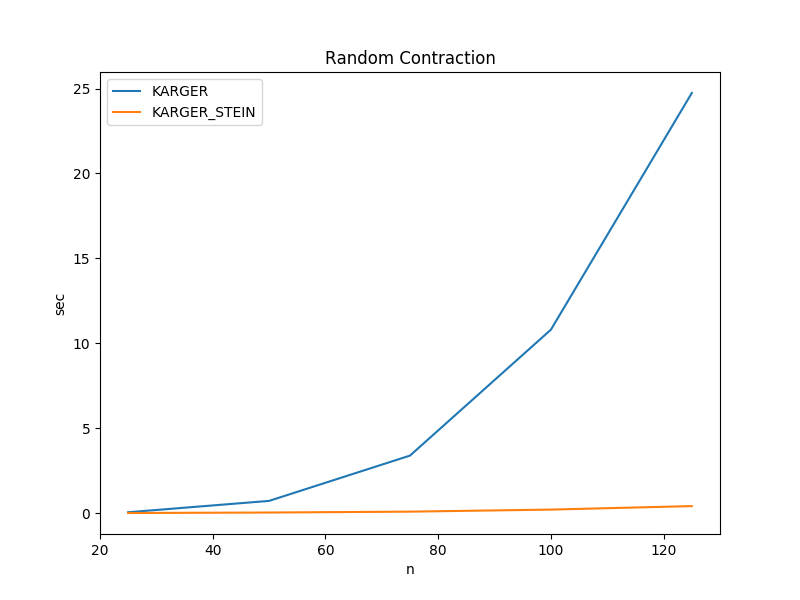The actual run times for finding the minimum cut of a randomly generated graph are shown below.

## Random ContractionALGORITHM n=25,m=250 n=50,m=500 n=75,m=750 n=100,m=1000 n=125,m=1250
KARGER 0.053415 sec 0.722489 sec 3.389049 sec 10.802166 sec 24.735355 sec
KARGER_STEIN 0.009551 sec 0.037323 sec 0.089343 sec 0.210117 sec 0.416360 sec

Key Takeaways:

• A probabilistic algorithm can be run multiple times to achieve a higher probability of success.
• By increasing the probability of producing the correct answer on each iteration, the total number of iterations is decreased. This results in HUGE overall run time savings.

Full Repo

Relevant Files: